# C Program to check Perfect Square

Perfect Square:
In mathematics, a square number, sometimes also called a perfect square, is an integer that is the square of an integer;  in other words, it is the product of some integer with itself. For example, 9 is a square number, since it can be written as 3?×?3. (Text from Wikipedia)
We are going to write a program that checks if the input number is a perfect square or not.
C program to check Perfect Square:

```/** C Program to check Perfect Square **/

#include <stdio.h>

int main()
{
int a, n;
printf("Enter a number: ");
scanf("%d", &n);
for(a = 0; a <= n; a++)
{
if (n == a * a)
{
printf("YES");
return 0;
}
}
printf("NO");
return 0;
}```

C Program to check Perfect Square using sqrt() function:
We can perform the same program getting help from sqrt() function. The sqrt() function is bundled with math.h header file. Here is the program:

```/* C Program to check Perfect Square using sqrt function */

#include <stdio.h>
#include <math.h>

int main(
{
int n, temp;
printf("Enter a number: ");
scanf("%d",&n);

temp = sqrt(n);

if(temp*temp == n) printf("YES.");
else printf("NO.");

return 0;
}
```
Share:

#### 28 comments :

1.In first method, please put two printf function before ("YES"); and ("NO"); otherwise it will print nothing.

1.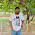Thanks for inidicating my mistake. Post is updated now.

2.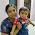if i try this and we i execute a square number it shows "YESNO"
both printf are executed what shall i do or use any other loop huh?

3.@Madhan Mafiya, There is a statement return 0; after printf("YES");. So if the number is a perfect square then the program will exit right after printing YES.

Or you may track with an extra variable. Look at this example:
#include

int main()
{
int a, n, perfectSquare;
printf("Enter a number: ");
scanf("%d", &n);
perfectSquare = 0;
for(a = 0; a <= n; a++)
{
if (n == a * a)
{
perfectSquare = 1;
break;
}
}
if(perfectSquare) printf("YES");
else printf("NO");
return 0;
}

2.#include
#include

int main(void)

{
int number;
int k;
double result;

number = 6400;

result= sqrt (number);
k = sqrt (number);
if (result - k == 0)
printf ("\n The integer HAS a perfect square \n\n");

else
printf ("\n The integer DOES NOT HAVE a perfect square \n\n");

return 0;
}

1.2.3.4.so simple programme... thnx for helping me....

5.what if I need to enter a number more than one time ?
I used while loop but I can't organize the sequence of my statements
help

1.Have a look at this:

#include "stdio.h"

int main()
{
int a, n;

do
{
printf("Enter a number (0 to exit): ");
scanf("%d", &n);
for(a = 0; a <= n/2; a++)
{
if (n == a * a)
{
printf("YES, %d is a perfect square\n", n);
break;
}
}
printf("NO, %d is not a perfect square\n",n);
}while(n!=0);
return 0;
}

2./* This is the syntax of C */
/* In C by default return type of main function is void*/
/* So it is not necessary to return a value */
#include
main()
{
int a, n;

do
{
printf("\nEnter a number to find is it a perfect number or not. And 0 to exit : ");
scanf("%d",&n);
for(a=0;a<= n/2; a++)
{
if (n== a * a)
{
printf("\nYES, %d is a perfect square\n", n);
break;
}
}

printf("\nNO, %d is not a perfect square\n",n);

}while(n!=0);
}

6.we have one more optimised code to check whether the given number is perfect square or not

isPerfectSquare(int n)
{
int i=1;

while(n>0)
{
n - =i;
i=i+2;
}

if(n==0)
return 1;
else return 0;

}

this above function tests whether the given number is perfect square or not.

try to test it and let me know if there are any mistakes found

7.how we can enter into the loop before knowing the value of a????

8.Write a C++ program to nd the square root of a perfect square. The program should
ask the user to enter a positive interger which is a perfect square number and then the
program should compute the square root and display the solution.?? please help meh with this question

9.Write a C++ program to nd the square root of a perfect square. The program should
ask the user to enter a positive interger which is a perfect square number and then the
program should compute the square root and display the solution. please help me with this.

10./** C Program to check Perfect Square **/

#include

int main()
{
int a, n;
printf("Enter a number: ");
scanf("%d", &n);
for(a = 0; a <= n; a++)
{
if (n == a * a)
{
printf(" The perfect square of given number is %d",a);
return 0;
}
}
printf("Not a perfect Square number");
return 0;

11.int isquare(long long n)
{
return !(sqrt(n)-int(sqrt(n)));
}
the function returns 1 if the number is perfect square.
else 0.

12.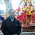#include
#include
void main()
{
int n,d;
clrscr();
printf("\nEnter Any Number:- ");
scanf("%d",&n);
for(d=1;d<=n;d++)
{
if(n/d==d && n%d==0)
{
printf("%d is perfect square of %d.",n,d);
goto RAJ;
}
}
printf("%d is not perfect square.",n);
RAJ:
getch();

}

13.14.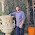//there are two ways of finding whether the number is perfect square or not
//We all know that all perfect squares always end in 1,4,5,6,9, or an even number of zeroes. And we have also noticed that for a number that ends in 1,4,9 its tens digit will always be even (2,4,6,8,0). If it ends with 6, its tens digit will be odd (1,3,5,7,9). If it ends with 5, its tens digit will be 2.
//this will make whole thing very easier!!

bool check_even_zeros(int number){
int count=0;

while(number!=0){
if(number%10!=0){
break;
}

if(number%10==0){
count=count+1;
}
number=number/10;
}
if(count>0 && count%2==0){
return true;
}
else return false;
}

bool not_a_perfect_square_checker(int number){

int k=(number/10)%10;

if(number==0){
return false;
}

else if(number%10==0){

if(check_even_zeros(number)==true) return true;
else false;
}

else if(number%10==2 || number%10==3 || number%10==7 || number%10==8)
{
return false;
}

else if(number%10==1 || number%10==4 || number%10==9){

if(k%2==0) return true;
else return false;
}

else if(number%10==6){
if((k%2)!=0) return true;
else return false;
}

else if(number%10==5){
if(k==2) return true;
else return false;
}

else{
return true;

}

}

15.http://thehacker123.blogspot.in/2015/07/perfect-square-there-are-two-ways-of.html
check this out!!

1.http://www.mycodingland.com/2015/10/display-numbers-and-their-squares-in-cpp-programming.html

16.Thanks bro, good also see my blog :
http://www.mycodingland.com/2015/10/display-numbers-and-their-squares-in-cpp-programming.html

17.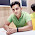C++ program to find Square Root of a Number

Thanks for sharing this code. It's really helpful. Keep sharing.

18.write a java program to print all 4digit no which is it self a perfect sqare and 1st two and last two digit also perfect sqare ? Plz reply the answer

19.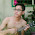can any one help me to solve this problem about perfect square.,,.,.,
,.,.hers the question,,,

create a that will ask the user to input a positive whole number. And the program should then display perfect square. if the number provided by the user is indeed a perfect square ; other wise , NOT PERFECT SQUARE , However, in implimentatoin of your program ,you cannot use any built-in function c .it needs a sulotion /algorithm in determining if the number is perfect square. lastly ,trap all possible input erros.,.

.,.,pls help.,.

20.write a program to display the square of an entered number if the number is even and cube if the number is odd

Spam comments will be deleted. :)